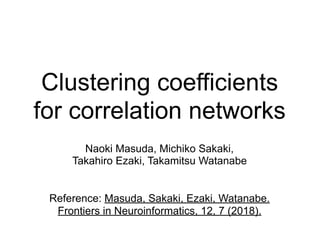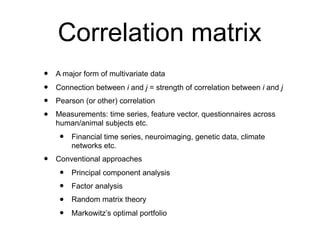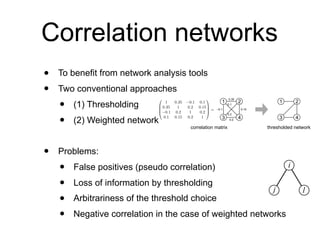Successfully reported this slideshow.

# Clustering coefficients for correlation networks

0

Share×
1 of 14
1 of 14

# Clustering coefficients for correlation networks

0

Share

Presentation slides for
Naoki Masuda, Michiko Sakaki, Takahiro Ezaki, Takamitsu Watanabe.
Frontiers in Neuroinformatics, 12, 7 (2018).

Presentation slides for
Naoki Masuda, Michiko Sakaki, Takahiro Ezaki, Takamitsu Watanabe.
Frontiers in Neuroinformatics, 12, 7 (2018).

## More Related Content

### Related Books

Free with a 14 day trial from Scribd

See all

### Related Audiobooks

Free with a 14 day trial from Scribd

See all

### Clustering coefficients for correlation networks

1. 1. Clustering coefficients for correlation networks Naoki Masuda, Michiko Sakaki, Takahiro Ezaki, Takamitsu Watanabe Reference: Masuda, Sakaki, Ezaki, Watanabe. Frontiers in Neuroinformatics, 12, 7 (2018).
2. 2. Correlation matrix • A major form of multivariate data • Connection between i and j = strength of correlation between i and j • Pearson (or other) correlation • Measurements: time series, feature vector, questionnaires across human/animal subjects etc. • Financial time series, neuroimaging, genetic data, climate networks etc. • Conventional approaches • Principal component analysis • Factor analysis • Random matrix theory • Markowitz’s optimal portfolio
3. 3. Correlation networks • To benefit from network analysis tools • Two conventional approaches • (1) Thresholding • (2) Weighted networks • Problems: • False positives (pseudo correlation) • Loss of information by thresholding • Arbitrariness of the threshold choice • Negative correlation in the case of weighted networks 0.2 for EPSRC corrmat -0.1 0.35 0.15 0.1 0.2 Figure 1: Thresholding of a correlation matrix into a network. A threshold of 0.12 is applied as an example. A different threshold value yields a different network in general. 1 2 3 4 1 2 3 4 0 B B @ 1 0.35 0.1 0.1 0.35 1 0.2 0.15 0.1 0.2 1 0.2 0.1 0.15 0.2 1 1 C C A = correlation matrix thresholded network i lj
4. 4. Aim • Less biased analysis methods for correlation networks • For community detection, see: MacMahon & Garlaschelli, Physical Review X, 5, 021006, 2015. • We do this for the clustering coefficient in this study.
5. 5. False positive problem in the clustering coefficient • If nodes i and j are correlated and i and ℓ are correlated, j and ℓ would be as well. • The problem persists for weighted networks. • Clustering coefficient tends to be inflated if we apply conventional unweighted/weighted networks to correlation matrices (Zalesky et al., 2012) i lj
6. 6. Idea • Use the partial correlation (3 ways) • We don’t use the partial correlation matrix (N ways) to make our clustering coefficient a local quantity (like the conventional clustering coefficients). i lj ⇢partial (j, ` | i) = ⇢(j, `) ⇢(i, j)⇢(i, `) p 1 ⇢2(i, j) p 1 ⇢2(i, `)
7. 7. Def 1 Local clustering coefficient at node i Global: Note: We use the absolute value of the partial cor. Ccor,A i = P 1j<`N j,`6=i ⇢(i, j)⇢(i, `)⇢partial (j, ` | i) P 1j<`N j,`6=i |⇢(i, j)⇢(i, `)| Ccor,A = 1 N NX i=1 Ccor,A i ⇢partial (j, ` | i) = ⇢(j, `) ⇢(i, j)⇢(i, `) p 1 ⇢2(i, j) p 1 ⇢2(i, `)
8. 8. Def 2 For multivariate Gaussian, where Ccor,M = 1 N NX i=1 Ccor,M i Ccor,M i = P 1j<`N j,`6=i |⇢(i, j)⇢(i, `)| ⇥ I(Xj, X` | Xi) 1+ln 2⇡ 2 P 1j<`N j,`6=i |⇢(i, j)⇢(i, `)| . I(Xj, X` | Xi) = h(Xj, Xi) + h(X`, Xi) h(Xi) h(Xj, X`, Xi) h(X↵1 , . . . , X↵d ) = d 2 (1 + ln 2⇡) + 1 2 ln det cov0 cov0 ij = E ⇥ X↵i X↵j ⇤ I(Xj, X` | Xi) = 1 2 ⇥ ln 1 ⇢2 (i, j) + ln 1 ⇢2 (i, `) ln 1 ⇢2 (i, j) ⇢2 (i, `) ⇢2 (j, `) + 2⇢(i, j)⇢(i, `)⇢(j, `) ⇤ Partial mutual information Then, simplify Local Global
9. 9. Numerical illustrations • Data: functional magnetic resonance imaging (fMRI) data (brain activity) • Nathan Klein Institute’s Rockland Sample • open resource • 18-85 years, healthy • N=30 nodes using a famous brain atlas (Power et al., 2011) • Biological interest: age dependence
10. 10. r = -0.377 r = -0.311r = 0.020 r = -0.397
11. 11. Effect of node strength (s+) regressed out r = -0.224 r = -0.259 (P = 0.0076) (P = 0.0019)
12. 12. Local clustering coef vs node strenegth Weighted clustering coefsOur clustering coefs i lj
13. 13. Other results • More correlated with the age than conventional unweighted/weighted clustering coefficients • Partial correlation matrix and then conventional clustering coefficients does not help. • Two other similar definitions of clustering coefficients. • Qualitatively the same results
14. 14. Conclusions • Network analysis of correlation matrices • Two clustering coefficients tailored to correlation matrices • Future: • Other networks (e.g. financial, genetic) • Different brain networks/contexts • Ref: Masuda, Sakaki, Ezaki, Watanabe. Frontiers in Neuroinformatics, 12, 7 (2018).